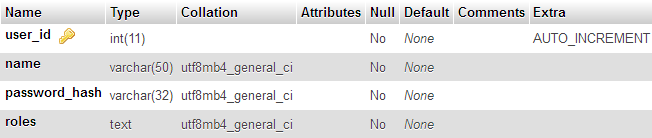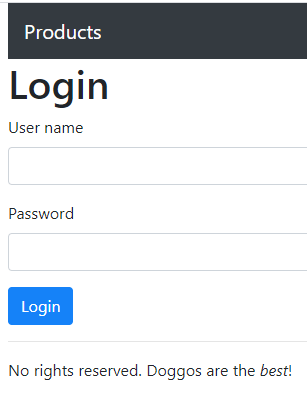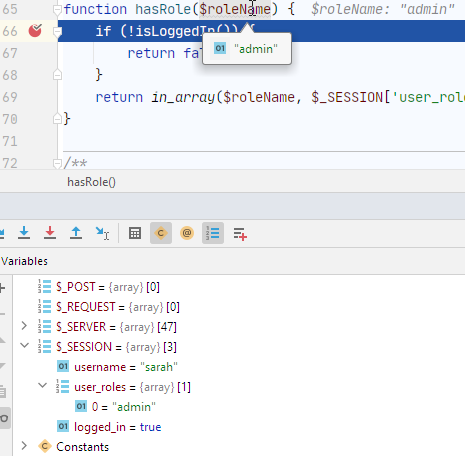Tags

### Where are we?

The code to check permission, and change menus, relies on two functions:

• `isLoggedIn()`: returns true if the user is logged in
• `hasRole(\$roleToCheck)`: returns true if the user has the role

With these two functions, we can make a simple user login system.

The two functions use data from the session to do their thing.

#### `isLoggedIn()`

`isLoggedIn()` relies on `\$_SESSION['logged_in']`, a boolean:

• /**
•  * Is the current user logged in?
•  * @return bool True if logged in, else false.
•  */
• function isLoggedIn() {
•     \$loggedIn = false;
•     if (isset(\$_SESSION['logged_in'])) {
•         if (\$_SESSION['logged_in']) {
•             \$loggedIn = true;
•         }
•     }
•     return \$loggedIn;
• }

The code uses the flag pattern.

Pattern

Use a variable as a flag. Set the flag if any of a number of things happens. After, check the flag to see if any of those things happened.

#### `hasRole(\$roleToCheck)`

`hasRole(\$roleToCheck)` relies on `\$_SESSION['user_roles']` to do its work.

• /**
•  * Does the current user have the given role?
•  * @param string \$roleName Role name, using consts above.
•  * @return bool True if has role, else false.
•  */
• function hasRole(string \$roleName) {
•     if (!isLoggedIn()) {
•         return false;
•     }
•     return in_array(\$roleName, \$_SESSION['user_roles']);
• }

#### Users table

We have a DB table with user names, password hashes, and roles.Here's the basic logic for login:

•   If they match:
•     Put data into the session

Code on pages we want to protect will check the data in the session.

How to implement logout? The easiest way is to destroy the session.

### The form

Here's what the form should look like:It's a Bootstrap form, of course.

Earlier, you learned how to have form input and validation on the same page. Let's do that again.

Pattern

Put a form and its validation code on the same page.

### The code

Here it is.

1. <?php
2. require_once 'library/useful-stuff.php';
4. if (isLoggedIn()) {
6.     exit();
7. }
8. \$errorMessage = '';
9. // Load default values for widgets.
12. // Is there post data?
13. if (\$_POST) {
14.     // User filled in data and sent it to this page.
15.     // Grab the data sent.
18.     // Is it valid?
20.     if (\$success) {
23.         exit();
24.     }
25.     // Show an error message.
26.     \$errorMessage = 'Sorry, login failed.';
27. }
28. ?><!doctype html>
29. <html lang="en">
30.   ...
32.   <?php
33.   // If there's an error message, show it.
34.   if (\$errorMessage != '') {
36.   }
37.   ?>
38.   <form method="post">
39.       <div class="mb-3">
43.           >
44.       </div>
45.       <div class="mb-3">
49.           >
50.       </div>
52.   </form>
53.   ...

Let's check it out. If the user is already logged in, then they don't need to log in again, so we can leave.

1. if (isLoggedIn()) {
3.     exit();
4. }

Notice how easy to understand the code is. The function `isLoggedIn()` is true when... well, when the user is logged in. Set up and name your functions well, and your code is easy to understand, easy to debug, and easy to modify. That's three easys!

Init `\$errorMessage`, and variables representing values for the widgets. Part of the same-page validation.

• \$errorMessage = '';
• // Load default values for widgets.

If there's POST data, put it into the variables we just made, and check it.

1. if (\$_POST) {
2.     // User filled in data and sent it to this page.
3.     // Grab the data sent.
6.     // Is it valid?
8.     if (\$success) {
11.         exit();
12.     }
13.     // Show an error message.
14.     \$errorMessage = 'Sorry, login failed.';
15. }

Line 19 is:

`logIn()` is a new function, in the library. We send it the user name and password from the form. It returns either true, or false. It also stores the right data in the session.

If `logIn()` returns true, we jump to the home page. If not, the program continues, and shows the form again, but with an error message above it:

• <?php
• // If there's an error message, show it.
• if (\$errorMessage != '') {
• }
• ?>
• <form method="post">
•   ...
• </form>

### `logIn()`

Here's some pseudocode for the function.

• Check the DB for a user record with the username and hashed password
• If it isn't there:
• Put user data into the session

Here's the code, from the library.

1. /**
4.  * @return bool True if login succeeded, else false.
5.  */
7.     global \$dbConnection;
10.     // Exit if either is MT or null.
13.        return false;
14.     }
17.     /** @var PDO \$dbConnection */
18.     \$stmnt = \$dbConnection->prepare(\$sql);
19.     \$isQueryWorked = \$stmnt->execute([
22.     ]);
23.     if (! \$isQueryWorked ) {
24.         // Query did not work.
25.         return false;
26.     }
27.     if (\$stmnt->rowCount() != 1) {
29.         return false;
30.     }
31.     \$userEntity = \$stmnt->fetch();
32.     // Store data in session.
34.     \$_SESSION['user_roles'] = explode(',', \$userEntity['roles']);
35.     \$_SESSION['logged_in'] = true;
36.     return true;
37. }

3.     // Exit if either is MT or null.
6.        return false;
7.     }Oh, that's cool. The username and password are both trimmed, so if someone hits the spacebar after typing them in, it won't matter.

Right! Another reason there might be an extra space, is if the user copy-and-pasted, and didn't get the text selection quite right. It's easy to accidentally include a space.

The first validation (lines 11 to 14) is both fields are required. I could have made the test simpler, but I tested for both MT and null, just in case.

We're up to:

• UP TO HERE
• Check the DB for a user record with the username and hashed password
• If it isn't there:
• Put user data into the session

Now, let's check to see if the username and password are in the DB. Actually, we know the password is not in the DB, because we don't store passwords. We store hashes, instead. So:

3.     /** @var PDO \$dbConnection */
4.     \$stmnt = \$dbConnection->prepare(\$sql);
5.     \$isQueryWorked = \$stmnt->execute([
8.     ]);
9.     if (! \$isQueryWorked ) {
10.         // Query did not work.
11.         return false;
12.     }
13.     if (\$stmnt->rowCount() != 1) {
15.         return false;
16.     }
17.     \$userEntity = \$stmnt->fetch();Ethan

So the code doesn't know whether it was the password that was wrong, or the username. Or even both.

Right! It doesn't need to know, since it wouldn't tell the user what was bad anyway. The whole login works, or the whole login doesn't.

OK, we're up to:

• Check the DB for a user record with the username and hashed password
• If it isn't there:
• UP TO HERE
• Put user data into the session

Last bit:

1. \$userEntity = \$stmnt->fetch();
2. // Store data in session.
4. \$_SESSION['user_roles'] = explode(',', \$userEntity['roles']);
5. \$_SESSION['logged_in'] = true;
6. return true;

The user's data is in `\$userEntity`, the record fetched from the database.

Line 35 sets `\$_SESSION['logged_in']`. That's what `isLoggedIn()` checks:

• /**
•  * Is the current user logged in?
•  * @return bool True if logged in, else false.
•  */
• function isLoggedIn() {
•     \$loggedIn = false;
•     if (isset(\$_SESSION['logged_in'])) {
•         if (\$_SESSION['logged_in']) {
•             \$loggedIn = true;
•         }
•     }
•     return \$loggedIn;
• }

`\$_SESSION['user_roles']` is used in `hasRole(\$roleToCheck)`:

• /**
•  * Does the current user have the given role?
•  * @param string \$roleName Role name, using consts above.
•  * @return bool True if has role, else false.
•  */
• function hasRole(string \$roleName) {
•     if (!isLoggedIn()) {
•         return false;
•     }
•     return in_array(\$roleName, \$_SESSION['user_roles']);
• }

Remember, we want `\$_SESSION['user_roles']` to be an array, with an entry for each role the user has. That's an easy way to let users have more than one role. Then, we can ask whether the role we want to check (`\$roleName`) is in the array:

• return in_array(\$roleName, \$_SESSION['user_roles']);

Here's what the debugger shows when checking for a role:You can see that `\$_SESSION['user_roles']` is an array, with an element for each role the user has. There's just one element in the array here, but there could be more than one.

When we were making the users table, we decided to put the roles in a text field. If someone has more than one role, we'd put something like this in the roles field: `admin,manager`. That is, we'd separate the role names with commas.

We want to make `admin,manager` into the array, with the first item as `admin`, and the second as `manager`. How to do that? like this:

• \$_SESSION['user_roles'] = explode(',', \$userEntity['roles']);

`explode(separator,string)` chops up a string, using the separator, and puts the pieces in array elements. For example,...

`explode(' ', 'Doggos are great!')`

... would return an array with three elements...

` => 'Doggos',  => 'are',  => 'great!'`

If `\$userEntity['roles']` is `'admin,manager'`, then...

`explode(',', \$userEntity['roles'])`

... would return an array with two elements...

` => 'admin',  => 'manager'`

### Logout

The functions `isLoggedIn()` and `hasRole(\$roleToCheck)` rely on session variables. For example:

• /**
•  * Is the current user logged in?
•  * @return bool True if logged in, else false.
•  */
• function isLoggedIn() {
•     \$loggedIn = false;
•     if (isset(\$_SESSION['logged_in'])) {
•         if (\$_SESSION['logged_in']) {
•             \$loggedIn = true;
•         }
•     }
•     return \$loggedIn;
• }

If `\$_SESSION['logged_in']` isn't there, `isLoggedIn()` returns false. So does `hasRole(\$roleToCheck)`.

So, an easy way to log someone out is to destroy the session. This is `logout.php`, the whole thing:

• <?php
• require_once 'library/useful-stuff.php';
• logOut();

Here's `logOut()`, from the library:

• /**
•  * Logout.
•  */
• function logOut() {
•     session_destroy();
•     exit();
• }

`session_destroy()` wipes out the session variables, all of them. That's all you need to do!

### Exercise

Exercise

Wombat user accounts

Earlier, you wrote an app to "list wombats:/exercise/wombat-list. Add user accounts to it, like the product app.

• Redirect non-logged in users to a login page.
• Add `isLoggedIn()` and `hasRole()` functions in your library.
• A `users` table in your database.